Next: Field Due to a Up: Relativity and Electromagnetism Previous: Transformation of Fields

# Potential Due to a Moving Charge

Suppose that a particle carrying a chargemoves with uniform velocity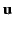through a frame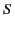. Let us evaluate the vector potential,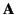, and the scalar potential,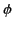, due to this charge at a given event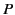in.

Let us choose coordinates inso that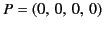and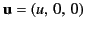. Let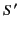be that frame in the standard configuration with respect toin which the charge is (permanently) at rest at (say) the point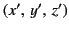. In, the potential atis the usual potential due to a stationary charge,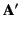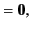(1813)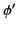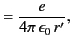(1814)

where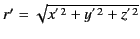. Let us now transform these equations directly into the frame. Because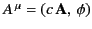is a contravariant 4-vector, its components transform according to the standard rules (1694)-(1697). Thus,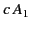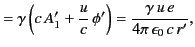(1815)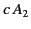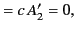(1816)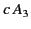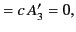(1817)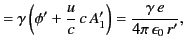(1818)

because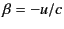in this case. It remains to express the quantity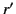in terms of quantities measured in. The most physically meaningful way of doing this is to expressin terms of retarded values in. Consider the retarded event at the charge for which, by definition,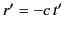and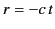. Using the standard Lorentz transformation, (1643)-(1646), we find that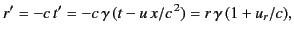(1819)

where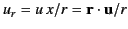denotes the radial velocity of the change in. We can now rewrite Equations (1817)-(1820) in the form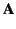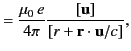(1820)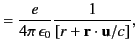(1821)

where the square brackets, as usual, indicate that the enclosed quantities must be retarded. For a uniformly moving charge, the retardation ofis, of course, superfluous. However, because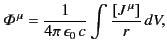(1822)

it is clear that the potentials depend only on the (retarded) velocity of the charge, and not on its acceleration. Consequently, the expressions (1822) and (1823) give the correct potentials for an arbitrarily moving charge. They are known as the Liénard-Wiechert potentials.Next: Field Due to a Up: Relativity and Electromagnetism Previous: Transformation of Fields
Richard Fitzpatrick 2014-06-27